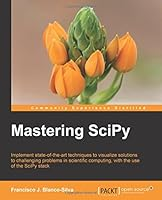# Mastering SciPy## Book Description

Implement state-of-the-art techniques to visualize solutions to challenging problems in scientific computing, with the use of the SciPy stack About This Book Master the theory and algorithms behind numerical recipes and how they can be applied to real-world problems Learn to combine the most appropriate built-in functions from the SciPy stack by understanding the connection between the sources of your problem, volume of data, or computer architecture A comprehensive coverage of all the mathematical techniques needed to solve the presented topics, with a discussion of the relevant algorithms built in the SciPy stack Who This Book Is For If you are a mathematician, engineer, or computer scientist with a proficiency in and familiarity with IPython, this is the book for you. Some basic knowledge of numerical methods in scientific computing would be helpful. What You Will Learn Master relevant algorithms used in symbolic or numerical mathematics to address approximation, interpolation, differentiation, integration, root-finding, and optimization of scalar or multi-variate functions Develop different algorithms and strategies to efficiently store and manipulate large matrices of data, in particular to solve systems of linear equations, or compute their eigenvalues/eigenvectors Understand how to model physical problems with systems of differential equations and distinguish the factors that dictate the strategies to solve them Perform statistical analysis, hypothesis test design and resolution, or at a higher level, and apply them to real-life problems in the field of Gain insights on the power of distances, Delaunay triangulations and Voronoi diagrams for Computational Geometry, and apply them to various engineering problems Familiarize yourself with different techniques in /image , including filtering audio, images, or video to extract information, features, or remove

Chapter 1. Numerical Linear Algebra
Chapter 2. Interpolation and Approximation
Chapter 3. Differentiation and Integration
Chapter 4. Nonlinear Equations and Optimization
Chapter 5. Initial Value Problems for Ordinary Differential Equations
Chapter 6. Computational Geometry
Chapter 7. Descriptive
Chapter 8. Inference and Data Analysis
Chapter 9. Mathematical Imaging

## Book Details

• Title: Mastering SciPy
• Author:
• Length: 363 pages
• Edition: 1
• Language: English
• Publisher:
• Publication Date: 2016-01-01
• ISBN-10: 1783984740
• ISBN-13: 9781783984749